## Extended model for capacity utilization & inflation

I have been in the workshop building a model for inflation and other things. It builds upon the model for forecasting the profit rate cycle. And also upon the model for the relationship between inflation and net profit rates. The model was provoked by a video from Khan Academy on youtube.

This model has many working parts so I will build it slowly.

## Effective demand limit & Peak profit rates

When profit rates peak in the aggregate, we have reached the effective demand limit. Capital utilization has been optimized. Think of the effective demand limit as the Natural limit of the business cycle in terms of capacity utilization, not full employment of labor.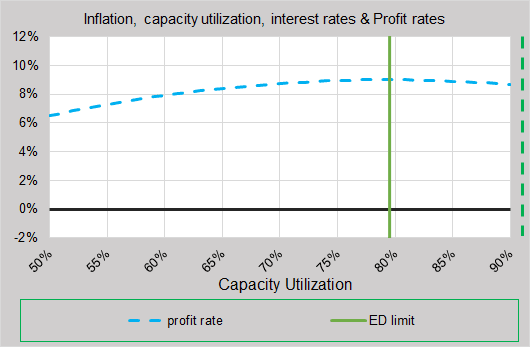Equations…

• Profit rate = 0.344*(c + u – (1.457 – s)*c2 u2)/s – 0.524
• Effective demand upon capacity utilization = s/u = 79.4%
• c = capacity utilization
• u = (1 – unemployment rate), unemployment rate of 5.6% used for graph
• s = effective labor share, (0.76 * Non-farm labor share index), s = 75% for graph.

Capacity is optimized at the effective demand limit. Capacity utilization does not like to go beyond the effective demand limit because profit rates fall. This is the pattern since 1967, the year data for capacity utilization starts.

## Estimated Fed Funds Rate

Now I add in the equation for estimating the Fed funds rate path based on the effective demand limit.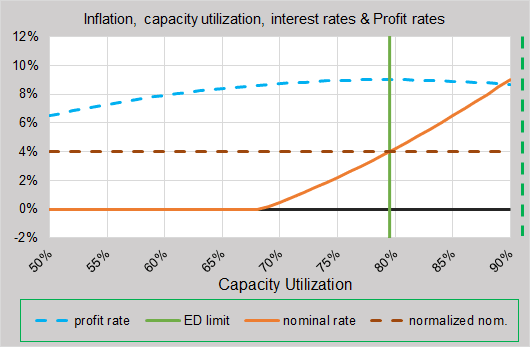Equations…

• Effective Demand Fed funds rate estimation = z(c2 u2 + s2 ) – (1 – z)(cu + s) + t
• z = (2s + r*)/(2s + 2s2)

• t = inflation target, 2% used for graph
• r* = natural real rate, 2% used for graph

The orange line sets an appropriate rate for the Fed funds rate based on the cycle of effective demand. However, that rate can change as the forces around inflation change. I will discuss this a bit later below.

Note how the orange line crosses the effective demand limit at 4%, which is the natural real rate plus the inflation target. So the Fed rate is modeled to be normalized at the effective demand limit. I have drawn in the normalized Fed rate line at 4%.

## Net Profit Rate

Now I add in the net profit rate.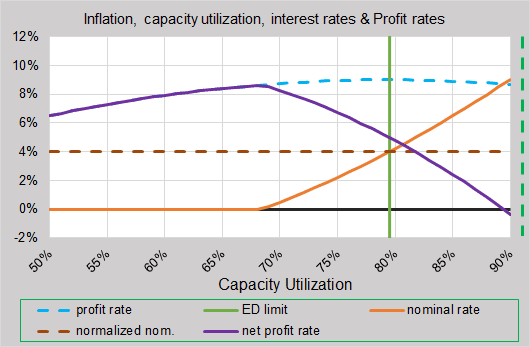Equation…

• Net profit rate, n = Profit rate – nominal rate

Net profit rate drops as the Fed rate rises.

## Inflation

Now I add in the trend of core inflation.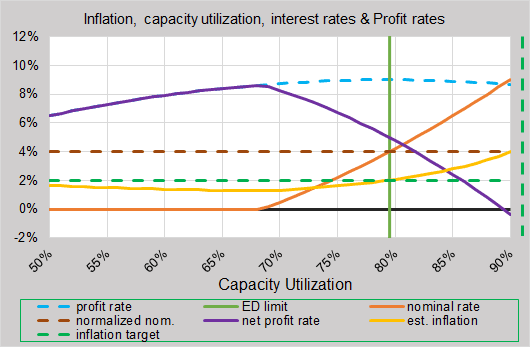Equation…

• Estimated core inflation, i = 0.038 * e(-12.7*n)

Inflation is estimated from the net profit rate, n. See this post for example of equation. The coefficients above were chosen to weed out some of the effect of the Volcker recession and to make inflation cross the effective demand limit at the inflation target of 2%.

Note that inflation is less than the 2% target to the left of the effective demand limit. The equation above for the estimated Fed rate assumes that inflation is on target at 2% because no adjustment is made in the equation for inflation off target. The implication is that there is a tendency for inflation to want to go below target when capacity utilization is low and when net profits are high.

The main point of the video by Khan Academy is that inflation tends to rise when capacity utilization is high. I would add that net profit rates need to be low too. The other point in the video is that inflation will stay low when capacity utilization is low. I would also add that net profits need to be high.

Here is how the graph changes just after two iterations if the Fed rate tries to adjust to the tendencies of inflation to be off target.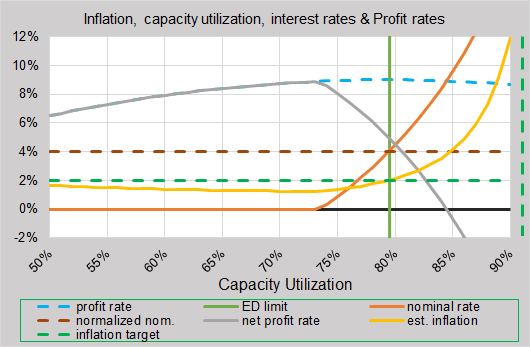First, note how the Fed rate waits longer to start rising off of the zero lower bound. Look familiar? Then it has to rise faster to normalize.

Inflation drops just a bit when capacity utilization is below optimal, and rises much faster when beyond optimal. The current stubborn low inflation reflects this model.

Many people criticize the Fed for keeping the Fed rate too low as a recovery gets going, but there is a reason to their madness. They are dealing with forces that try to push inflation lower.  Especially now since capacity utilization is very low and net profits are very high. Both add to pressure to keep inflation low. The problem with the Fed is simply that they do not know where the effective demand limit is.

## Real Profit Rate

More important than the net profit rate is the real profit rate. Firms look at this in order to invest, set prices and manage their profits.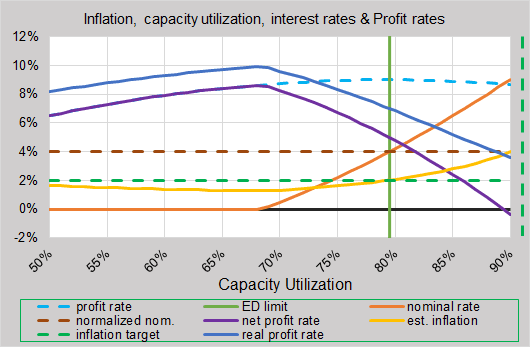Equation…

• Real profit rate = n + i = net profit rate + core inflation

Firms keep the real profit rate above zero. The aggregate real profit rate does not like to go below zero. So you see it dropping toward zero beyond the effective demand limit.

Here is the same graph above adding the real profit rate when the Fed rate tries to adjust to strong forces pushing inflation off target.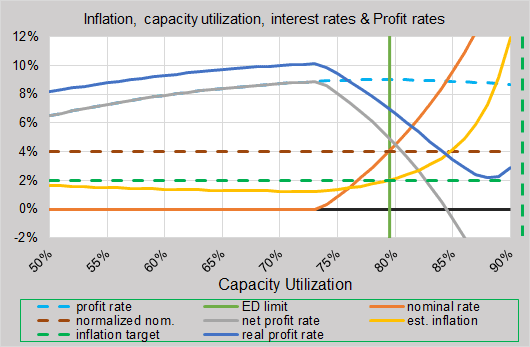The real profit rate will drop faster around the effective demand limit as the Fed rate rises faster. Then inflation will rise faster on the right of the ED limit to keep the real profit rate positive. In the graph, the real profit rate slams on the brakes and starts rising again as inflation keeps it above zero. Aggregate profit rates are still dropping, but an immature economy may simply keep increasing capacity utilization as long as the real profit rate is positive. The result is high inflation.

## Conclusion

The model extends the view of the forces pushing inflation off target. The foundation of the model is the cycle of the aggregate profit rate.

Comments Off on Extended model for capacity utilization & inflation | |